# Energy Stored in an Electric Field

Energy Stored in an Electric Field

Work must be done by an external agent to charge a capacitor. Starting with an uncharged capacitor, for example, imagine that-using “magic tweezers”-you remove electrons from one plate and transfer them one at a time to the other plate. The electric field that builds up in the space between the plates has a direction that tends to oppose further transfer. Thus, as charge accumulates on the capacitor plates,  ou have to do increasingly larger amounts of work to transfer additional electrons. In practice. this work is done not by “magic tweezers” but by a battery, at the expense of its store of chemical energy  We visualize the work required to charge a capacitor as being stored in the form  of electric potential energy U in the electric field between the plates. You can recover this energy at will, by discharging the capacitor in a circuit. just as you can recover the potential energy stored in a stretched bow by releasing the  bowstring to  t ansfer the energy to the kinetic energy of an arrow.Suppose that, at a given instant, a charge q’ has been transferred from one plate of a capacitor to the other. The potential difference V’ between the plates at that.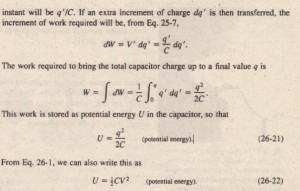Equations 26-21 and 26·22 hold no matter what the geometry of the capacitor is. To gain some physical insight into energy storage, consider two parallel- plate capacitors that are identical except that capacitor 1 has twice the plate separation of capacitor 2. Then capacitor 1 has twice the volume between its plates and also, from , half the capacitance of capacitor 2. Equation 26-4 tells us that if both capacitors have the same charge q, the electric fields between their plates are identical. And Eq. 26-21 tells us that capacitor 1 has twice the stored potential energy of  capacitor 2. Thus, of two otherwise identical capacitors with the same charge and same electric field, the one with twice the volume between its plates has twice the stored potential energy. Arguments like this tend to verify our earlier assumption:

The Medical Deftbrillator

The ability of a capacitor to store potential energy is the basis of defibrillator devices, which are used by emergency medical teams to stop the fibrillation of heart attack victims. In the portable version, a battery charges a capacitor to a high potential difference, storing a large amount of energy in less than a minute. The battery maintains only a modest potential difference; an electronic circuit repeatedly uses that potential difference to greatly increase the potential difference of the capacitor. The. power, or rate of energy transfer. during this process is also modest. Conducting leads (“paddles”) are placed on the victim’s chest. When a control switch is closed, the capacitor sends a portion of its stored energy from paddle to paddle through the victim. As an example, when a 70 /LF capacitor in a defibrillator is charged to 5000 V, Eq. 26-22 gives the energy stored in the capacitor as.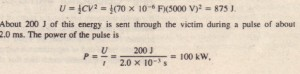which is much greater than the power of the battery itself. This same technique of slowly charging a capacitor with a battery and then discharging the capacitor at a much higher power is commonly used in flash photography and stroboscopic photography.

Energy Density

In a parallel-plate capacitor, neglecting fringing, the electric field has the same value at all points between the plates. Thus, the energy density u-that is, the potential energy per unit volume between the plates-should also be uniform. We can find II by dividing the total potential energy by the volume Ad of the space between the plates. Using Eq. 26-22, we obtain.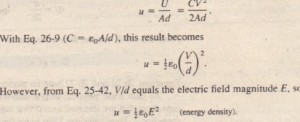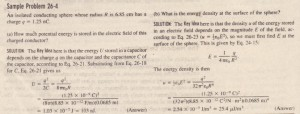Capacitor with a Dielectric

If you fill the space between the plates of a capacitor with a dielectric, which is an insulating material such as mineral oil or plastic, what happens to the capacitance? Michael Faraday-to whom the whole concept of capacitance is largely due and for whom the SI unit of capacitance is named – first looked into this matter in 1837. Using simple equipment much like that shown in  he found that the .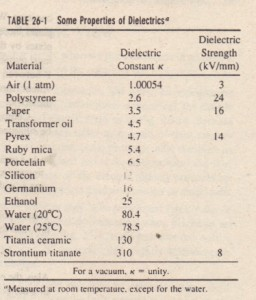capacitance increased by a numerical factor K, which he called the dielectric constant of the insulating material. Table 26-1 shows some dielectric materials and their dielectric constants. The dielectric constant of a vacuum is unity by definition. Because air is mostly empty space, its measured dielectric constant is only slightly greater than unity.  Another effect of the introduction of a dielectric is to limit the potential difference that can be applied between the plates to a certain value Vmax• called the breakdown potential. If this value is substantially exceeded  the dielectric material will break down and form a conducting path between the plates. Every dielectric material has a characteristic dielectric strength. which is the maximum value of the electric field that it can tolerate without breakdown. A few such values are listed in Table  6-1.As we discussed in connection with Eq. 26-18, the capacitance of any capacitor can be written in the form.

C = ec:J;£,

In which £ has the dimensions of a length. For example  = Aid for a parallel plate capacitor. Faraday’s discovery was that, with a dielectric completely filling the space between the plates, Eq. 26-24 becomes where Cairo is the value of the capacitance with only air between the plates.  provides some insight into Faraday’s experiments. In  the battery ensures that the potential difference V between the plates will remain.